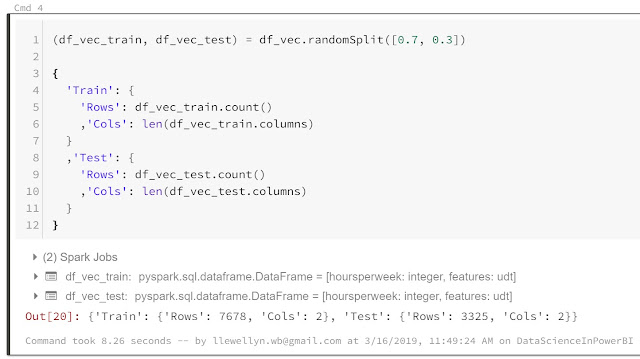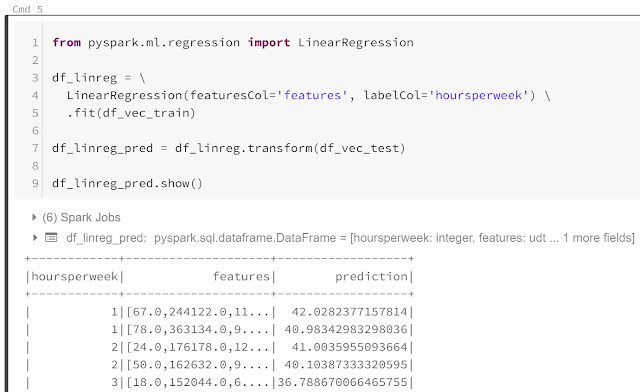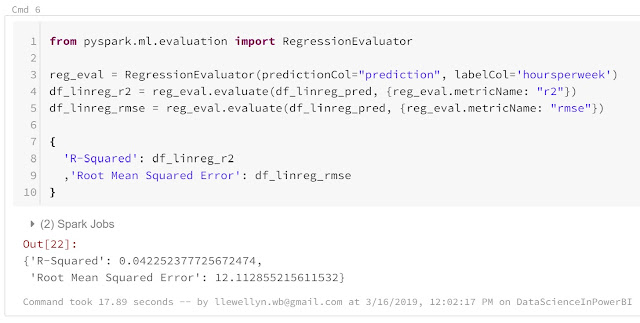## Monday, April 22, 2019

### Data Science In Power BI: Spark MLlib

Today, we're going to talk about Spark MLlib within Power BI.  If you haven't read the earlier posts in this series, IntroductionGetting Started with R ScriptsClusteringTime Series DecompositionForecastingCorrelationsCustom R VisualsR Scripts in Query EditorPythonAzure Machine Learning StudioStream AnalyticsStream Analytics with Azure Machine Learning StudioHDInsight Hive and Databricks Spark, they may provide some useful context.  You can find the files from this post in our GitHub Repository.  Let's move on to the core of this post, Spark MLlib.

Technically, we won't be running Spark MLlib commands within Power BI in this post.  There's probably a way to pull that off using the built-in Python functionality.  Alas, that's a bit more complex than we'd like to get into here.  Instead, we're going to use Spark MLlib functionality within Databricks to generate some predictions for us, then expose those predictions using Power BI.  So, what's Spark MLlib?

MLlib is one of the primary extensions of Spark, along with Spark SQL, Spark Streaming and GraphX.  It is a machine learning framework built from the ground up to be massively scalable and operate within Spark.  This makes it an excellent choice for machine learning applications that need to crunch extremely large amounts of data.  You can read more about Spark MLlib here.

In order to leverage Spark MLlib, we obviously need a way to execute Spark code.  In our minds, there's no better tool for this than Azure Databricks.  In the previous post, we covered the creation of an Azure Databricks environment.  We're going to reuse that environment for this post as well.  We'll also use the same dataset that we've been using, which contains information about individual customers.  This dataset was originally designed to predict Income based on a number of factors.  However, we left the income out of this dataset a few posts back for reasons that were important then.  So, we're actually going to use this dataset to predict "Hours Per Week" instead.

Spoiler: The resulting model is terrible, but it lets us show off some cool functionality.

To accomplish this, we're going to use Pyspark.  Pyspark is a Python library that allows us to execute Spark commands using Python.  Given the meteoric rise of Python as a Data Engineering and Data Science Programming, as well it's ease of coding when compared to Scala, we find it to be a great option for Spark programming.  You can read more about Pyspark here.Import Data
<CODE START>

df = sqlContext.read.parquet("/mnt/datadefaultparquet")

display(df)

<CODE END>

The first step is to import our data.  Since we previously mounted the Parquet data, this is a relatively simple use of "sqlContext".  The MLlib functionality that we are going to leverage today doesn't like strings very much.  In a real-world use case, we would use dummy encoding, also known as indicator variables and one-hot processing, to solve this problem.  We've covered this process using Azure Machine Learning Studio in this post.  It's not a basic task in PySpark and deserves its own post.  So, we're just going to remove all of the string columns.Clean Data
<CODE START>

df_clean = df.drop('customerid', 'workclass', 'education', 'maritalstatus', 'occupation', 'relationship', 'race', 'sex', 'nativecountry')

display(df_clean)

<CODE END>

Now that our dataset is cleaned up and ready for modelling, we need to "vectorize" it.  Basically, MLlib doesn't like having multiple feature columns.  Instead, it wants us to provide a single "vector" that contains all of our features.  This is a requirement driven by the linear mathematics behind-the-scenes.  However, we're not sure why PySpark requires this, as it seems easy enough for it create on its own without our help.  Alas, it is a requirement nonetheless.  Fortunately, there's a built-in function to handle it.Vectorize Features
<CODE START>

from pyspark.ml.feature import VectorAssembler

df_vec = \
VectorAssembler( \
inputCols=[ \
c \
for c in df_clean.columns \
if c != 'hoursperweek' \
] \
,outputCol="features" \
) \
.transform(df_clean) \
.select(['hoursperweek', 'features'])

df_vec.show()

<CODE END>

First, we have to import the "VectorAssembler()" function from the "pyspark.ml.feature" library.  We don't need to install these libraries on the cluster, as they come prepackaged with Databricks clusters.  However, we still need to import them into our notebooks.  Then, we create a new "df_vec" data frame by telling the "Vector Assembler" to vectorize all features EXCEPT "hoursperweek" into a single column called "features".  We then see the result of this operation.

There are a few important syntactical notes here.  First, PySpark executes commands when it sees a line break.  So, to clean up our code using multiple lines, we have to add a "\" to the end of the line.  This tells PySpark that the command continues to the next line.  Also, the "display()" function displays some strangeness when we apply it to vectors.  So, we switched over to the ".show()" function.

Next, we need to create training and testing splits of our data.Split Data
<CODE START>

(df_vec_train, df_vec_test) = df_vec.randomSplit([0.7, 0.3])

{
'Train': {
'Rows': df_vec_train.count()
,'Cols': len(df_vec_train.columns)
}
,'Test': {
'Rows': df_vec_test.count()
,'Cols': len(df_vec_test.columns)
}

}

<CODE END>

We can use the built-in ".randomSplit()" function to achieve this.  This is one of the interesting Python functions that outputs multiple data frames at once.  Therefore, we need to capture it using the "(df1, df2)" syntax.  Finally, we can showcase the important aspects of these data frames by creating a dictionary, which uses the "{}" syntax.  We can see that data frames both have two columns, while the training data frame has many more observations than the testing data frame due to the 70/30 split.  Next, we can train our linear regression model.Linear Regression
<CODE START>

from pyspark.ml.regression import LinearRegression

df_linreg = \
LinearRegression(featuresCol='features', labelCol='hoursperweek') \
.fit(df_vec_train)

df_linreg_pred = df_linreg.transform(df_vec_test)

df_linreg_pred.show()

<CODE END>

To accomplish this, we have to import another function, "LinearRegression()", this time from the "pyspark.ml.regression" library.  One of the interesting aspects of PySpark that took us some time to understand is that PySpark leverages a "lazy" execution model.  This means that commands do not run until there is some need for them to provide output.  This allows some interesting coding options.  Notice the "LinearRegression(featuresCol='features', labelCol='hoursperweek')" command that we run here.  This command is not tied to a dataset, meaning that it can't possibly return a result.  It is simply an empty mathematical formula waiting for a dataset to use it on.  However, it's still completely valid PySpark because, as we said, it isn't executed until it's needed.  So, once we apply the ".fit(df_vec_train)" function, it has a data set and can begin doing it's magic.  The lazy execution model is actually more complex than this, but this is a decent example to showcase it.

Moving on, now that we have predictions from our linear regression model, we can calculate our evaluation metrics to see how good our model is.  BRACE YOURSELVES!Model Evaluation
<CODE START>

from pyspark.ml.evaluation import RegressionEvaluator

reg_eval = RegressionEvaluator(predictionCol="prediction", labelCol='hoursperweek')
df_linreg_r2 = reg_eval.evaluate(df_linreg_pred, {reg_eval.metricName: "r2"})
df_linreg_rmse = reg_eval.evaluate(df_linreg_pred, {reg_eval.metricName: "rmse"})

{
'R-Squared': df_linreg_r2
,'Root Mean Squared Error': df_linreg_rmse

}

<CODE END>

Wow....that is one terrible model.  If an R-Squared of 4% isn't a record low, then it must be close.  Regardless, this was a showcase of functionality given an incomplete dataset.  We were able to calculate these values using the "RegressionEvaluator()" function from "pyspark.ml.evaluation" library.

Finally, we need to store these results in the Databricks database for access from Power BI.Predictions
<CODE START>

df_linreg_pred.write.saveAsTable("Predictions")

<CODE END>

<CODE START>

%sql

SELECT * FROM Predictions LIMIT 10;

<CODE END>

Now that our data is stored in the Predictions table, we can access it from Power BI.  As in the previous post, you can find the connection walkthrough here.Power BI Report
This quick scatterplot of our predictions versus the actual values tells the same story as our R-Squared of 4%.  If our model was perfect, these points would all fall exactly on the line.  As an aside, this line set to "y=x" is called a "Ratio Line" in Power BI.

Hopefully, this post piqued your interest in combining the massive computing power of Spark MLlib with the ease of use and reporting capabilities of Power BI.  This blog series has been very interesting to write and we've learned a ton along the way.  We're not sure what the next series will be, but we hope you'll tune in!  Thanks for reading.  We hope you found this informative.

Brad Llewellyn
Service Engineer - FastTrack for Azure
Microsoft
@BreakingBI
www.linkedin.com/in/bradllewellyn
llewellyn.wb@gmail.com